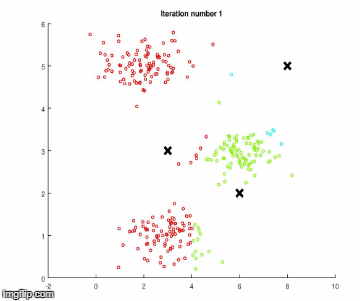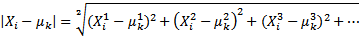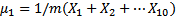Over a million developers have joined DZone.{{announcement.body}}
{{announcement.title}}

# Java Machine Learning for Image Color Reduction

DZone 's Guide to

# Java Machine Learning for Image Color Reduction

### Learn how to use k-means to train a program that automatically reduces the size of images to make them more optimized without sacrificing quality.

· AI Zone ·
Free Resource

Comment (1)

Save
{{ articles.views | formatCount}} Views

K-means is one of the most famous and widely used algorithms in machine learning. In this post, we're going to use k-means to reduce colors on images (without reducing pixels) and therefore reduce their size, as well. No knowledge in this field is required, and a friendly user interface is already provided by the executable application file (150MB because of long Spark dependencies). You can easily experiment on your own with different images. The full working code is available on GitHub.

## K-Means Algorithm

K-means is an unsupervised learning algorithm that classifies similar data into categories/clusters. It is unsupervised because the data are not labeled and the algorithm does not need feedback for categorizing similar data together (just maybe the number of expected categories — more on this later).

### Applications

Some applications of the k-means algorithm include customer focus, organizing computing in clusters, social networks, and astronomical data analysis.

#### Customer Focus

Say you have a large amount of data related to your customers and want to learn more about the type of customers you have so that you can to develop your business better in serving specific groups. Maybe you want to produce jeans and T-shirts and you want to group people in a certain country by size so that you know what sizes will fit better in the population.

#### Organize Computing in Clusters

It's better from a performance perspective to group certain computers in clusters together; for example, those that exchange often, are close from a network perspective, or serve similar computations. K-means can group similar computers in clusters together so we can developer better layouts and optimizations.

#### Social Networks

In social networks, you can group people by a lot of factors — their relationships, preferences, similarities, etc. — and target them better from a marketing point-of-view. Based on the input of data we give, k-means can help us categorize the same data from different perspectives.

#### Astronomical Data Analysis

K-means is also used in understanding galaxy formation and searching for cohesion in astronomical data.

### How It Works

K-means operates in two steps. Let's say we want to categorize our data into four groups. We'll perform the following steps.

Note: Before starting any of the steps, k-means randomly picks three samples from the data, known as cluster centroids.

1. It checks each of the data samples and categorizes them into groups depending on how similar they are to the cluster centroids randomly chosen at the beginning.

2. It moves cluster centroids a little bit closer to their similar counterparts (categorized at Step 1).

It repeats these steps until there is no significant movement of cluster centroids. Below is an execution of the algorithm with simple data.Source

#### More on Step 1

Let's get a more formal explanation of how Step 1 is implemented. If you're not familiar with multidimensional feature data, please see here.

• k: Number of clusters

• Xij: Example i with feature j

• μij: Cluster centroid i with feature (similar to example X because the centroid was just a randomly picked example)

At this step, we iterate through examples, calculate how similar they are to the centroids (we have one centroid for category), and put them in the category they fit into. More formally, this is done by calculating the Euclidean distance of the example from the centroid and picking the centroid from which we have the smallest distance. Since the centroid is just a random example, we sum all features of the Euclidean distance between centroids and each example.Or, more simplified and with less computation...#### Step 2

Graphically, this step is just moving centroids a little closer to the similar examples categorized in Step 1. More formally, we calculate the new position of each centroid by taking the average of all examples similar to them or belonging to them (categorized by Step 1).

For example, if we have four clusters and 103 examples after Step 1, suppose we have the following outcome:

• μ1=20 examples categorized similar to centroid 1 from index 1 to 20

• μ2=10 examples categorized similar to centroid 2 from index 21 to 31

• μ3=30 examples categorized similar to centroid 3 from index 32 to 62

• μ4=40 examples categorized similar to centroid 4 from index 63 to 103

The new centroids will be calculated as follows:Basically, this is an average calculation of all data similar to a particular centroid.

#### Repeat, Repeat, Repeat... When to Stop?

We repeat Step 1 and Step 2 with newly calculated centroids until, graphically, the centroids move closer and closer to the clusters of data. The algorithm will run forever and we need to explicitly tell it when we are satisfied with the result so that it can stop. One way to tell that is when iteration-by-iteration, the centroids are not moving anymore in the graph, or they're moving very little. Formally, we can calculate the cost function, which is basically the average of what we did in Step 1:μc is the centroid for the particular Xi example; as we know, each example can be part of different groups or centroids. Each iteration cost is compared to the previous cost. If the change is really low, then we stop. For example, we can stop if the improvement (difference of cost functions) is 0.00001 (or any other value we find suitable) and it makes no sense to continue anymore.

#### Can It Go Wrong?

Usually not, but it is known that k-means canget stuck at local optima instead of global optimum. In that case, k-means fails to discover even obvious groups, as shown in the below graph:Fortunately, the solution is fairly easy — just run k-means several times and pick the best result. This solution helps because at the very beginning, we randomly initialize k-means and it's very rare to run, let's say, 10 times, and have all local optima. Of course, this increases the running time, as it runs several times and only one result is needed. On the other hand, it's totally possible to run on parallel or even different clusters, so usually, it's fairly acceptable as a working solution.

Of course, there is more to k-means than what I've covered, so I highly recommend this article for more deep insight.

## Algorithm Execution and Results

In this section, let's execute the running application (also possible through downloading the code) and see the results with some details how k-means helps with color reduction.

### Color Reduction

It's important to clarify that k-means is not reducing the pixels on the image but rather is reducing the number of colors an image has by grouping similar colors together. A normal image usually has thousands or even more colors, so reducing the number of colors can dramatically decrease file size.

To clarify how reducing the number of colors helps with reducing image size, let's look at an example. Suppose we have an image of 1280x1024 pixels, and for each pixel, there's a simple color representation (RGB 24 bit, 8-bit red, 8-bit green, 8-bit blue). In total, we need 1280 * 1024 * 24 = 31.457.280 bits or 30MB to represent the image. Now, let's say we reduce the overall colors to 16. This means that we do not need 24-bit for each pixel but 4-bit to represent only 16 colors. Now, we have 1280 * 1024 * 4 = 5MB — which is 6x less memory. Of course, our image will not look as perfect as it did before (it has only 16 colors now), but we can certainly find a middle ground.

### Execution and Results

The easiest way to execute the algorithm on is to download the JAR and execute with your own images (you need Java installed on your machine). It takes my computer (depending on the image, of course) approximately one minute to reduce colors to 16 (expect high CPU and memory usage since Spark is running in parallel). In the user interface, you can choose the image file you want to try and also the numbers of color to reduce the image to. Below is an example of the user interface and results:As we can notice, the file size is reduced by a factor of four, and the final image doesn't look that bad. Running several times it may bring better results. Let's try again with 24 colors:This looks noticeably better and the size is not increased much (just 0.08MB). It seems that maybe between 24 and 28 is the best visual result for this image.

Although the results look quite good, choosing the best image is a manual task. After all, we are executing and picking what looks best to the eye.

I believe that this problem can be solved in a number of different ways.

One of the solutions would be simple to count all colors on the original image and from there, define the number of colors used for the image to still look good. This process can be done by using a machine learning prediction algorithm like linear regression. We train the algorithm by giving images with different colors and, at the same time, say for each of them what looks good. After giving significant examples like this, the algorithm learns pretty much the best color to reduce to, depending on different images. Now is the time to let the linear regression algorithm predict for the next image how much the colors will be reduced by.

It is possible to download the code and run it on a Java IDE through executing the class. Or, if you do not feel to run from the source, simply download the code and run maven: `mvn clean install exec:java`.

Topics:
machine learning ,ai ,tutorial ,k-means ,unsupervised learning ,clusters ,data analytics ,java ,spark mlib ,image processing

Comment (1)

Save
{{ articles.views | formatCount}} Views

Opinions expressed by DZone contributors are their own.